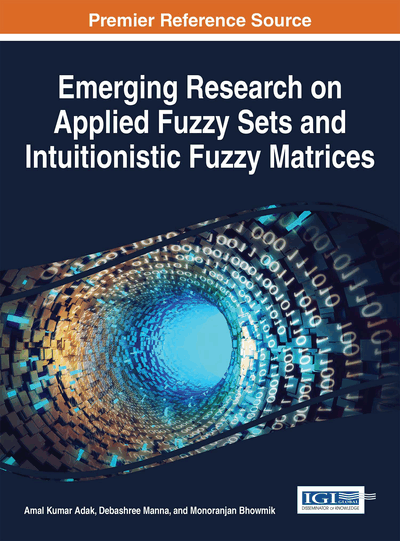# Decision Making by Using Intuitionistic Fuzzy Rough Set

T. K. Das
DOI: 10.4018/978-1-5225-0914-1.ch012
OnDemand:
(Individual Chapters)
Available
\$29.50
No Current Special Offers

## Abstract

This chapter begins with a brief introduction of the theory of rough set. Rough set is an intelligent technique for handling uncertainty aspect in the data. This theory has been hybridized by combining with many other mathematical theories. In recent years, much decision making on rough set theory has been extended by embedding the ideas of fuzzy sets, intuitionistic fuzzy sets and soft sets. In this chapter, the notions of fuzzy rough set and intuitionistic fuzzy rough (IFR) sets are defined, and its properties are studied. Thereafter rough set on two universal sets has been studied. In addition, intuitionistic fuzzy rough set on two universal sets has been extensively studied. Furthermore, we would like to give an application, which shows that intuitionistic fuzzy rough set on two universal sets can be successfully applied to decision making problems.
Chapter Preview
Top

## Foundations Of Rough Computing

At the present age of internet, a huge repository of the data is available across various domains. Therefore, it is very hard to extract useful information from voluminous data available in the universe. So, information retrieval and knowledge representation has become one of the most popular areas of recent research. Information retrieval and acquisition of knowledge is one of the important components of an information system. But, the real challenge lies in converting voluminous data into knowledge and to use this knowledge to make proper decisions. In order to transform the processed data into useful information and knowledge, there is a need of new techniques and tools. Rough set theory developed by Pawlak (1982) used to process uncertain and incomplete information is a tool to the above mentioned problem. One of its strength is the attribute dependencies, their significance among inconsistent data. At the same time, it does not need any preliminary or additional information about the data. Therefore, it classifies imprecise, uncertain or incomplete information expressed in terms of data acquired from experience.

### Rough Sets

In this section we recall the definitions of basic rough set theory developed by Pawlak (1991). Let U be a finite nonempty set called the universe. Supposeis an equivalence relation on U. The equivalence relation R partitions the set U into disjoint subsets. Elements of same equivalence class are said to be indistinguishable. Equivalence classes induced by R are called elementary concepts. Every union of elementary concepts is called a definable set. The empty set is considered to be a definable set, thus all the definable sets form a Boolean algebra and (U,R) is called an approximation space. Given a target set X, we can characterize X by a pair of lower and upper approximations. We associate two subsetsandcalled the R-lower and R-upper approximations of X respectively and are given by

The R-boundary of X, BNR(X) is given by. We say X is rough with respect to R if and only if, equivalently BNR(X)≠ϕ. X is said to be R- definable if and only ifor BNR(X)=ϕ. So, a set is rough with respect to R if and only if it is not R- definable.

## Complete Chapter List

Search this Book:
Reset